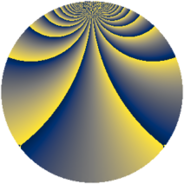# Properties

 Label 2280.2.cdLevel $2280$ Weight $2$ Character orbit 2280.cd Rep. character $\chi_{2280}(11,\cdot)$ Character field $\Q(\zeta_{6})$ Dimension $640$ Sturm bound $960$

# Related objects

## Defining parameters

 Level: $$N$$ $$=$$ $$2280 = 2^{3} \cdot 3 \cdot 5 \cdot 19$$ Weight: $$k$$ $$=$$ $$2$$ Character orbit: $$[\chi]$$ $$=$$ 2280.cd (of order $$6$$ and degree $$2$$) Character conductor: $$\operatorname{cond}(\chi)$$ $$=$$ $$456$$ Character field: $$\Q(\zeta_{6})$$ Sturm bound: $$960$$

## Dimensions

The following table gives the dimensions of various subspaces of $$M_{2}(2280, [\chi])$$.

Total New Old
Modular forms 976 640 336
Cusp forms 944 640 304
Eisenstein series 32 0 32

## Trace form

 $$640q + 2q^{4} + O(q^{10})$$ $$640q + 2q^{4} - 2q^{10} + 2q^{16} + 8q^{19} - 8q^{24} - 320q^{25} - 16q^{30} + 16q^{33} - 18q^{34} - 28q^{36} + 28q^{40} + 42q^{42} + 62q^{48} - 608q^{49} + 16q^{52} + 14q^{54} + 8q^{57} - 32q^{58} + 80q^{64} - 12q^{66} + 12q^{70} + 72q^{72} - 16q^{73} + 76q^{78} + 8q^{81} + 40q^{82} - 64q^{84} + 24q^{88} + 18q^{90} + 28q^{94} + 156q^{96} - 32q^{97} - 32q^{99} + O(q^{100})$$

## Decomposition of $$S_{2}^{\mathrm{new}}(2280, [\chi])$$ into newform subspaces

The newforms in this space have not yet been added to the LMFDB.

## Decomposition of $$S_{2}^{\mathrm{old}}(2280, [\chi])$$ into lower level spaces

$$S_{2}^{\mathrm{old}}(2280, [\chi]) \cong$$ $$S_{2}^{\mathrm{new}}(456, [\chi])$$$$^{\oplus 2}$$